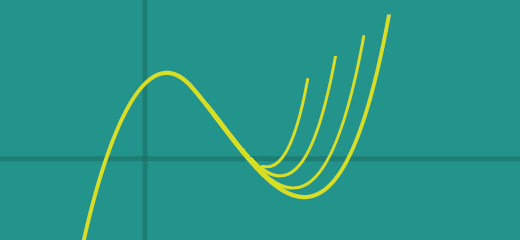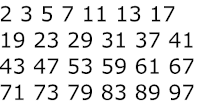# MT #2: Algebra

## Part A: Short-answer type questions

Submission file: Write answers to all the ten questions on a single sheet of paper. Email a picture of your answer sheet. Name the file as PartA.jpg.

For this part, answers must be written without any explanation.

1. Let $$p_1(x),p_2(x),\ldots,p_m(x)$$ be $$m$$ distinct polynomials such that:
$p_1(x) = p_2(x) = \ldots = p_m(x) \mbox{ if } x<0$ What is the largest possible value of $$m$$? For example, if it is possible for $$m$$ to be 4, then the polynomials might look like this:2. Find a polynomial $$q(x)$$ with integer coefficients with $$\sqrt{3}+i$$ as a root. In case there are multiple candidates, pick a polynomial with the least degree.

3. (i) Find the remainder when $$f(x)=6x^{16}+4x^{22}+5x^{12}+x^{2}$$ is divided by $$(x^{2}+1)$$. [2 marks]

(ii) Find the remainder when $$x f(x)$$ is divided by $$(x^{2}+1)$$. [2 marks]

4. Find two quadratic polynomials $$p(x)$$ and $$g(x)$$ that satisfy the following conditions:
1. Both $$p(x)$$ and $$g(x)$$ have two distinct real roots.
2. The sum $$p(x)+g(x)$$ has no real root.

5. A grazing field has $$10$$ kgs of grass. Every Sunday a herd of cows eats $$x$$ kgs of grass. Over a week's time the grass grows by $$10\%$$. What is the maximum value of $$x$$ that will allow the cows to feed indefinitely without running out of grass?

6. Consider the simultaneous equations in variables $$x$$ and $$y$$, where $$k$$ is a constant: \begin{align*} 2x + y &= kx + 4 \\ x + 2y &= ky + 6k \end{align*} For what values of $$k$$ does the system not have a solution?

7. Notation. $${ }^nC_{k}$$ represents the binomial coefficient.
Consider the set of prime numbers less than 100 (listed below). Pick two numbers $$n$$ and $$k$$ with $$n > k$$ from this set such that $${}^nC_{k}$$ is maximized.8. We have numbers $$x_1,\ldots,x_{51}$$ such that each $$x_i$$ is either $$+1$$ or $$-1$$. What is the minimum value of following sum? $\left\lvert \sum_{ 1\leq i < j \leq 51 } x_ix_j \right\rvert$

Problem source: PRMO 2019.

9. Notation. $$[n]$$ denotes the set of numbers $$\{1,2,\ldots,n\}$$. Assume $$n>100$$ for this problem.

A function $$f:[n]\rightarrow \mathbb{R}$$ is defined as follows:
$f(x) = \begin{cases} 0 &\mbox{if } x = 1 \\ 1 & \mbox{if } x = n \\ \frac{1}{2} (f(x-1) + f(x+1)) & \mbox{if } 1 < x < n \end{cases}$ What is the value of $$f(3)$$ in terms of $$n$$?

10. Let $$f(x) = 37 x^{4}-37 x^{3}-x^{2}+9 x-2$$. Let the four roots of $$f(x)$$ be $$r_{1}, r_{2}, r_{3}$$ and $$r_{4}$$. Find the value of $\left(r_{1}+r_{2}+r_{3}\right)\left(r_{1}+r_{2}+r_{4}\right)\left(r_{1}+r_{3}+r_{4}\right)\left(r_{2}+r_{3}+r_{4}\right)$ Problem source: Stanford Math Tournament.

## Part B: Subjective questions

Submission files: Each question in this part must be answered on a page of its own. Name the files as B1.jpg, B2.jpg, etc. In case you have multiple files for the same question, say B4, name the corresponding files as B4-1.jpg, B4-2.jpg, etc.

Clearly explain your entire reasoning. No credit will be given without reasoning. Partial solutions may get partial credit.

B1. Prove the following inequality for $$a, b>0$$: $\large \sqrt{a b^{2020}} \leq \frac{ a+2020b }{2021}$

Problem source: Problem solving strategies. Prob. 4, Inequalities.

B2. Consider the polynomial $$f(x) = a_{n}x^n +a_{n-1}x^{n-1} + \cdots + a_{1}x+a_{0}$$, where each coefficient $$a_{i}$$ is either $$0$$ or $$1$$. If $$f(2) = 14$$, find the polynomial $$f(x)$$.

B3. Compute the smallest value $$p$$ such that, for all $$q> p$$, the polynomial $$x^3 - 7x^2 +qx +16 = 0$$ has exactly one real root.

Problem source: Stanford Math Tournament.

B4. Let $$f(x)=a_{0} x^{n}+\cdots+a_{n}$$ be a non-constant polynomial with real coefficients satisfying $f(x) f\left(x^{2}\right)=f\left(x^{3}+x\right)$ for all real numbers $$x$$. Show that $$f$$ has no real root.

B5. Show that $$p(x)$$ does not have any real roots where: $p(x)=x^{2 n}-2 x^{2 n-1}+3 x^{2 n-2}-4 x^{2 n-3}+\cdots-2 n x+(2 n+1)$
Problem source: Problem solving strategies. Prob. 5, Polynomials.

B6. (i) Simplify $\sum_{j=0}^{n} \sum_{i=j}^{n}{ }^{n} C_{i}{ }^{i} C_{j}$

(ii) Calculate the value of the expression (i) when $$n=5$$.

Problem sources for B4 and B6: Madhava Mathematics Competition 2019.